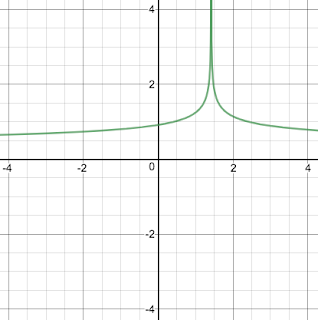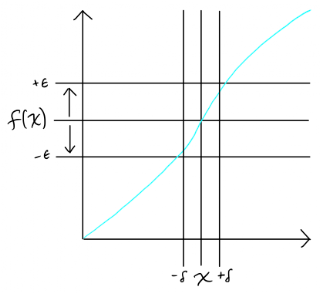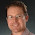# Continuous functions over the real numbers

As a high school student and undergraduate I learned in Calculus that
1. real numbers involve infinity in precision, e.g. some have no finite decimal representation, and
2. a continuous function forms an unbroken line, a necessary condition to be differentiable.
For an example, the decimal representation of $$\sqrt 2$$ goes on forever: $1.41421 \ldots$ Later on, in a course on Real Analysis, I learned that one way to define the real numbers is to declare them to be Cauchy sequences, that is, infinite sequences of rational numbers that get closer and closer together. So, for example, $$\sqrt 2$$ is declared to be the sequence $$1, \frac{3}{2}, \frac{17}{12}, \frac{577}{408}, \ldots$$ described by the following recursive formulas.
$A_0 = 1 \qquad A_{n+1} = \frac{A_n}{2} + \frac{1}{A_n} \hspace{1in} (1) \label{eq:caucy-sqrt-2}$
Depending on how close an approximation to $$\sqrt 2$$ you need, you can go further out in this sequence. (Alternatively, one can represent $$\sqrt 2$$ by its sequence of continued fractions.)
For an example of a continuous function, Figure 1 depicts $$x^3 - x^2 - 4x$$. On the other hand, Figures 2 and 3 depict functions that are not continuous. The function $$1/\mathrm{abs}(x-\sqrt 2)^{1/4}$$ in Figure 2 is not continuous because it goes to infinity as it approaches $$\sqrt 2$$. The function $$(x+1)\,\mathrm{sign}(x)$$ in Figure 3 is not continuous because it jumps from $$-1$$ to $$1$$ at $$0$$.Figure 1. The function $$x^3 - x^2 - 4x$$ is continuous.Figure 2. The function $$1/\mathrm{abs}(x-\sqrt 2)^{1/4}$$ is not continuous at $$\sqrt 2$$.Figure 3. The function $$(x+1)\,\mathrm{sign}(x)$$ is not continuous at $$0$$.

You may recall the $$\epsilon$$-$$\delta$$ definition of continuity, stated below and depicted in Figure 4.
A function $$f$$ is continuous at a point $$x$$ if for any $$\epsilon > 0$$ there exists a $$\delta > 0$$ such that for any $$x'$$ in the interval $$(x - \delta,x+\delta)$$, $$f(x')$$ is in $$(f(x) -\epsilon, f(x) + \epsilon)$$.
In other words, when a function is continuous, if you want to determine its result with an accuracy of $$\epsilon$$, you need to measure the input with an accuracy of $$\delta$$.Figure 4. The $$\epsilon$$-$$\delta$$ definition of continuity.
One connection between the infinite nature of real numbers and continuity that only recently sunk-in is that continuous functions are the ones that can be reasonably approximated by applying them to approximate, finitely-represented inputs. For example, suppose you wish to compute $$f(\sqrt 2)$$ for some continuous function $$f$$. You can accomplish this by applying $$f$$ to each rational number in the Cauchy sequence for $$\sqrt 2$$ until two subsequent results are closer than your desired accuracy. On the other hand, consider trying to approximate the function from Figure 2 by applying it to rational numbers in the Cauchy sequence for $$\sqrt 2$$. No matter how far down the sequence you go, you’ll still get a result that is wrong by an infinite margin!

# The $$\lambda$$-calculus and continuous functions

In graduate school I studied programming languages and learned that
1. the $$\lambda$$-calculus is a little language for creating and applying functions, and
2. Dana S. Scott’s semantics of the $$\lambda$$-calculus interprets $$\lambda$$’s as continuous functions.
For example, the $$\lambda$$ expression $\lambda x.\; x + 1$ creates an anonymous function that maps its input $$x$$, say a natural number, to the next greatest one. The graph of this function is $\left\{ \begin{array}{l} 0\mapsto 1, \\ 1\mapsto 2, \\ 2\mapsto 3, \\ \quad\,\vdots \end{array} \right\}$ which is infinite. So we have our first similarity between the real numbers and $$\lambda$$’s, both involve infinity.
A key characteristic of the $$\lambda$$-calculus is that functions can take functions as input. Thus, the semantics of the $$\lambda$$-calculus is also concerned with functions over infinite entities (just like functions over the real numbers). For example, here is a $$\lambda$$ expression that takes a function $$f$$ and produces a function that applies $$f$$ twice in succession to its input $$x$$. $\lambda f.\; \lambda x.\; f(f(x))$ The graph of this function is especially difficult to write down. Not only does it have an infinite domain and range, but each element in the domain and range is an infinite entity. $\left\{ \begin{array}{l} \{ 0\mapsto 1, 1\mapsto 2, 2\mapsto 3, \ldots \} \mapsto \{ 0\mapsto 2, 1\mapsto 3, 2\mapsto 4, \ldots \},\\ \{ 0\mapsto 0, 1\mapsto 2, 2\mapsto 4, \ldots \} \mapsto \{ 0\mapsto 0, 1\mapsto 4, 2\mapsto 8, \ldots \},\\ \ldots \end{array} \right\}$
Denotational semantics for the $$\lambda$$-calculus interpret $$\lambda$$’s as continuous functions, so just based on the terminology there should be another similarity with real numbers! However, these continuous functions are over special sets called domains, not real numbers, and the definition of continuity in this setting bears little resemblance to the $$\epsilon$$-$$\delta$$ definition. For example, in Dana S. Scott’s classic paper Data Types as Lattices, the domain is the powerset of the natural numbers, $$\mathcal{P}(\mathbb{N})$$. This domain can be used to represent a function's graph by encoding (create a bijection) between pairs and natural numbers, and between sets and naturals. The following are the easier-to-specify directions of the two bijections, the mapping from pairs to naturals and the mapping from naturals to sets of naturals.
\begin{aligned} \langle n, m \rangle &= 2^n (2m+1) - 1 \\ \mathsf{set}(0) &= \emptyset \\ \mathsf{set}(1+k) &= \{ m \} \cup \mathsf{set}(n) & \text{if } \langle n, m \rangle = k\end{aligned}
Scott defines the continuous functions on $$\mathcal{P}(\mathbb{N})$$ as those functions $$h$$ that satisfy
$h(f) = \bigcup \{ h(g) \mid g \subseteq_{\mathit{fin}} f \} \hspace{1in} (2) \label{eq:cont-pn}$
In other words, the value of a continuous function $$h$$ on some function $$f \in \mathcal{P}(\mathbb{N})$$ must be the same as the union of applying $$h$$ to all the finite subgraphs of $$f$$. One immediately wonders, why are the $$\lambda$$-definable functions continuous in this sense? Consider some $$\lambda$$ expression $$h$$ that takes as input a function $$f$$.
But $$f$$ is a function; an infinite object. What does it mean to “compute” with an “infinite” argument? In this case it means most simply that $$h(f)$$ is determined by asking of $$f$$ finitely many questions: $$f(m_0), f(m_1), ..., f(m_{k-1})$$. —Dana S. Scott, A type-theoretical alternative to ISWIM, CUCH, OWHY, 1969.
Put another way, if $$h$$ terminates and returns a result, then it will only have had a chance to call $$f$$ finitely many times. So it suffices to apply $$h$$ instead to a finite subset of the graph of $$f$$. However, we do not know up-front which subset of $$f$$ to use, but it certainly suffices to try all of them!

# Relating the two kinds of continuity

But what does equation (2) have to do with continuous functions over the real numbers? What does it have to do with the $$\epsilon$$-$$\delta$$ definition? This question has been in the back of my mind for some time, but only recently have I had the opportunity to learn the answer.
To understand how these two kinds of continuity are related, it helps to focus on the way that infinite entities can be approximated with finite ones in the two settings. We can approximate a real number with a rational interval. For example, refering back to the Cauchy sequence for $$\sqrt 2$$, equation (1), we have $\sqrt 2 \in \left(\frac{17}{12}, \frac{3}{2}\right)$ Of course an approximation does not uniquely identify the thing it approximates. So there are other real numbers in this interval, such as $$\sqrt{2.1}$$. $\sqrt{2.1} \in \left(\frac{17}{12}, \frac{3}{2}\right)$
Likewise we can approximate the infinite graph of a function with a finite part of its graph. For example, let $$G$$ be the a graph with just one input-output entry. $G=\{ 1 \mapsto 2 \}$ Then we consider $$G$$ to be an approximation of any function that agrees with $$G$$ (maps $$1$$ to $$2$$), which is to say its graph is a superset of $$G$$. So the set of all functions that are approximated by $$G$$ can be expressed with a set comprehension as follows: $$\{ f \mid G \subseteq f\}$$. In particular, the function $$+1$$ that adds one to its input is approximated by $$G$$. $\left\{ \begin{array}{l} 0\mapsto 1, \\ 1\mapsto 2, \\ 2\mapsto 3, \\ \quad\,\vdots \end{array} \right\} \in \{ f \mid G \subseteq f\}$ But also the function $$\times 2$$ that doubles its input is approximated by $$G$$. $\left\{ \begin{array}{l} 0\mapsto 0, \\ 1\mapsto 2, \\ 2\mapsto 4, \\ \quad\,\vdots \end{array} \right\} \in \{ f \mid G \subseteq f\}$ Of course, a better approximation such as $$G'=\{1\mapsto 2, 2\mapsto 3\}$$ is able to tell these two functions apart.
The interval $$(17/12, 3/2)$$ and the set $$\{f\mid G \subseteq f\}$$ are both examples of neighborhoods (aka. base elements) in a topological space. The field of Topology was created to study the essence of continuous functions, capturing the similarities and abstracting away the differences regarding how such functions work in different settings. A topological space is just some set $$X$$ together with a collection $$B$$ of neighborhoods, called a base, that must satisfy a few conditions that we won’t get into. We’ve already seen two topological spaces.
1. The real numbers form a topological space where each neighborhood consists of all the real numbers in a rational interval.
2. The powerset $$\mathcal{P}(\mathbb{N})$$ forms a topological space where each neighborhood consists of all the functions approximated by a finite graph.
The $$\epsilon$$-$$\delta$$ definition of continuity generalizes to topological spaces: instead of talking about intervals, it talks generically about neighborhoods. In the following, the interval $$(f(x) -\epsilon, f(x) + \epsilon)$$ is replaced by neighborhood $$E$$ and the interval $$(x - \delta,x+\delta)$$ is replaced by neighborhood $$D$$.
A function $$f$$ is continuous at a point $$x$$ if for any neighborhood $$E$$ that contains $$f(x)$$, there exists a neighborhood $$D$$ that contains $$x$$ such that for any $$y$$ in $$D$$, $$f(y)$$ is in $$E$$.
Now let us instantiate this topological definition of continuity into $$\mathcal{P}(\mathbb{N})$$.
A function $$f$$ over $$\mathcal{P}(\mathbb{N})$$ is continuous at $$X$$ if for any finite set $$E$$ such that $$E \subseteq f(X)$$, there exists a finite set $$D$$ with $$D \subseteq X$$ such that for any $$Y$$, $$D \subseteq Y$$ implies $$E \subseteq f(Y)$$.
Hmm, this still doesn’t match up with the definition of continuity in equation (2) but perhaps they are equivalent. Let us take the above as the definition and try to prove equation (2).
First we show that $h(f) \subseteq \bigcup \{ h(g) \mid g \subseteq_{\mathit{fin}} f \}$ Let $$x'$$ be an arbitrary element of $$h(f)$$. To show that $$x'$$ is in the right-hand side we need to identify some finite $$g$$ such that $$g \subseteq f$$ and $$x' \in h(g)$$, that is, $$\{x'\} \subseteq h(g)$$. But this is just what continuity gives us, taking $$h$$ as $$f$$, $$f$$ as $$X$$, $$\{x'\}$$ as $$E$$, $$g$$ as $$D$$, and also $$g$$ as $$Y$$. Second we need show that $\bigcup \{ h(g) \mid g \subseteq_{\mathit{fin}} f \} \subseteq h(f)$ This time let $$x'$$ be an element of $$\bigcup \{ h(g) \mid g \subseteq_{\mathit{fin}} f \}$$. So we known there is some finite set $$g$$ such that $$x' \in h(g)$$ and $$g \subseteq f$$. Of course $$\{x'\}$$ is a finite set and $$\{x'\} \subseteq h(g)$$, so we can apply the definition of continuity to obtain a finite set $$E$$ such that $$E \subseteq g$$ and for all $$Y$$, $$E \subseteq Y$$ implies $$\{x'\} \subseteq h(Y)$$. From $$E \subseteq g$$ and $$g \subseteq f$$ we transitively have $$E \subseteq f$$. So instantiating $$Y$$ with $$f$$ we have $$\{x'\} \subseteq h(f)$$ and therefore $$x' \in h(f)$$.
We have shown that the topologically-derived definition of continuity for $$\mathcal{P}(\mathbb{N})$$ implies the definition used in the semantics of the $$\lambda$$-calculus, i.e., equation (2). It is also straightforward to prove the other direction, taking equation (2) as given and proving that the topologically-derived definition holds. Thus, continuity for functions over real numbers really is similar to continuity for $$\lambda$$ functions, they are both instances of continuous functions in a topological space.

# Continuous functions over partial orders

In the context of Denotational Semantics, domains are often viewed as partial orders where the ordering $$g \sqsubseteq f$$ means that $$g$$ approximates $$f$$, or $$f$$ is more informative than $$g$$. The domain $$\mathcal{P}(\mathbb{N})$$ with set containment $$\subseteq$$ forms a partial order. Refering back to the examples in the first section, with $$G=\{ 1 \mapsto 2 \}$$ and $$G'=\{1\mapsto 2, 2\mapsto 3\}$$, we have $$G \sqsubseteq G'$$, $$G' \sqsubseteq +1$$, and $$G \sqsubseteq \times 2$$. In a partial order, the join $$x \sqcup y$$ of $$x$$ and $$y$$ is the least element that is greater than both $$x$$ and $$y$$. For the partial order on $$\mathcal{P}(\mathbb{N})$$, join corresponds to set union.
In the context of partial orders, continuity is defined with respect to infinite sequences of ever-better approximations: $f_0 \sqsubseteq f_1 \sqsubseteq f_2 \sqsubseteq \cdots$ A function $$h$$ is continuous if applying it to the join of the sequence is the same as applying it to each element of the sequence and then taking the join.
$h\left(\bigsqcup_{n\in\mathbb{N}} f_n\right) = \bigsqcup_{n\in\mathbb{N}} h(f_n) \hspace{1in} (3) \label{eq:cont-cpo}$
But this equation is not so different from the equation (2) that expresses continuity on $$\mathcal{P}(\mathbb{N})$$. For any function $$f$$ (with infinite domain) we can find an sequence $$(f_n)_{n=0}^{\infty}$$ of ever-better but still finite approximations of $$f$$ such that $f = \bigsqcup_{n\in\mathbb{N}} f_n$ Then both equation (2) and (3) tell us that $$h(f)$$ is equal to the union of applying $$h$$ to each $$f_n$$.

# Further Reading

The following is the list of resources that I found helpful in trying to understand the relationship between real numbers, $$\lambda$$’s, and the role of continuity.
• Data Types as Lattices by Dana S. Scott.
• A type-theoretical alternative to ISWIM, CUCH, OWHY by Dana S. Scott.
• The Formal Semantics of Programming Languages by Glynn Winskel.
• Topology via Logic by Steven Vickers.
• Topology (2nd Edition) by James R. Munkres.
• Introduction to Lattices and Order by B.A. Davey and H.A. Priestley.
• The Wikipedia articles on

#### 4 comments:

1.Very cool. I had not see the natural numbers as a topological space before. So I guess that continuous lambdas are the analogue of functions in constructive analysis?

1.I will need to read up on constructive analysis to answer your question!

2.Anonymous11:26 AM

This is very nice, thanks. You might want to revisit the "continuous functions are smooth and unbroken, which makes them differentiable" bit though :)

1.Thank you! I see that the sentence was mixing up what it means to be continuous and differentiable. I've revised.